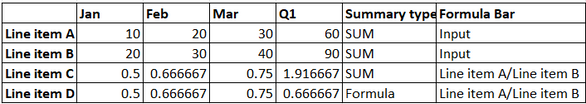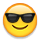# formula summaryHI All,

Why we use summary as formula.

what are the scenarios that we use this ?

what is the outcome of it?

•In simple words, When you use a formula logic in formula bar, you need to use formula as summary.

Below table should explain you.Thanks,
Manjunath

•As @ManjunathKN  was mentioning that we use formula as summary method, when there is formula present in the formula bar.

By doing this what happens is, the formula would also be applied to the summary items. Like in the example given by @ManjunathKN  for Q1 which is a summary of Jan, Feb, Mar, the formula(Line Item A/Line Itemis applied for Q1 also i.e  60/90=0.66.

Whereas in the other case the summary enabled as sum and it is just adding the result of Jan, Feb, Mar in the Line Item C (0.5+0.66+0.75=1.91)

Hope this helps

Thanks

Meda Ganesha Manikanta

Matasma Digital Technologies